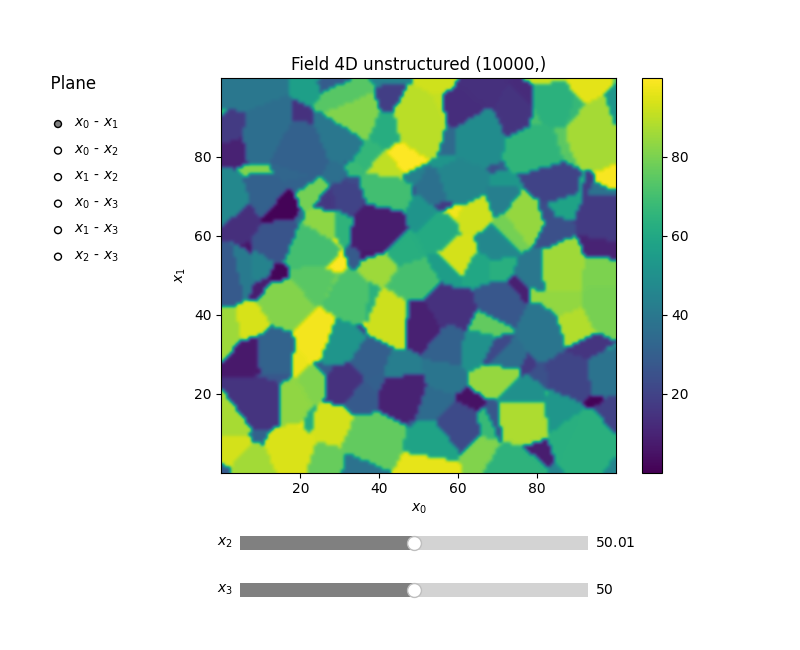# Standalone Field class

The `Field` class of GSTools can be used to plot arbitrary data in nD.

In the following example we will produce 10000 random points in 4D with random values and plot them.

```import numpy as np

import gstools as gs

rng = np.random.RandomState(19970221)
x0 = rng.rand(10000) * 100.0
x1 = rng.rand(10000) * 100.0
x2 = rng.rand(10000) * 100.0
x3 = rng.rand(10000) * 100.0
values = rng.rand(10000) * 100.0
```

Only thing needed to instantiate the Field is the dimension.

Afterwards we can call the instance like all other Fields (`SRF`, `Krige` or `CondSRF`), but with an additional field.

```plotter = gs.field.Field(dim=4)
plotter(pos=(x0, x1, x2, x3), field=values)
plotter.plot()
```Total running time of the script: ( 0 minutes 0.663 seconds)

Gallery generated by Sphinx-Gallery